# CSVTU Exam Question Papers-BE First-Year-Engineering Mechanics–Nov-Dec-2006

CSVTU Exam Question Papers-BE First-Year-Engineering Mechanics–Nov-Dec-2006

Examination- Nov-Dec 2006

Engineering Mechanics

UNIT- I

Q.1 (a) A barge is pulled by two tug boats . If the resultant of the tug boats is a 5000N force directed along the axis of the barge.

Determine graphically the value of a such that tension in rope AC is minimum . Also find the magnitude of forces on ropes A

and AB.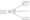(b) A hollow cylinder of radius r is open at both ends and rosts on a smooth horizontal plane. Two sphere having weights wand w2

and radial r1 and r2 respectively are placed inside the cylinder as shown . Find the minimum weight W of the cylinder in order

that it will not tip over. Neglect friction.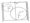(c) Two prismatic bar AB of length i and of negligible weight is hinged at A and supported at B by a string that posses over a pulley C .

A vertical load of W applied at the end of the B is supported by a force P applied to the string . Show that the compression in bar

AB is constant for all value of ?.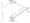UNIT- II

Q.2 (a) A cantilever truss is loaded and supported as shown . Find axial force of magnitude ken in the member AC. Also find the axial

force in member CD using method of section.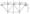(b) A truss is loaded and supported as shown in fig. 2.40 find the axial forces in the members BD, CD and CE(c) A beam AB is acted upon by the uniformly distributed load 2 kN/m and by two forces P and Q. It has experimental determine

that the bending moment is 172 kN —m at point and 235 kN -m at point E. Determine P and Q and find out bending moment just

be for point F.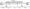UNIT- III

Q.3 (a) A wedge A of negligible weight is to be driven 40 kg plates B and C. The coefficient of s between all surfaces of contact is 0.35 .

Determine the magnitude of the force P required to start moving if plate C is securely bolted to the surface .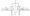(b) A pulley is driven by a flat belt . the angle of lap being 120? . The belt is 100 mm wide by 6 mm thick and density 1000 kg/ m3.

If the coefficient of friction is 0.3 and the maximum stress in the belt is not to exceed 2 Mpa. Find the greatest power which

the belt can transmit and corresponding speed of belt.

(c) Prove that the effieciency of self locked square screw is given by :

?? 1 – tan2 ?           where ?= efficiency
2         2                               ?=Friction

UNIT- IV

Q.4 (a) Find the moment of inertia of the area abstained after removing a semicircle of radius 10cm from a quadrant of a circle of

(b) Determine the product of inertia of a right angle triangle with respect to the Centro dial axes parallel to x and y — axes.(c) Determine the moment of inertia of a cylinder with respect to its longitudinal axis. Take L = length of the cylinder and r = radius

of cylinder .

Two blocks A and B are released from rest on a incline , when they are 18m apart . The coefficient of friction under the upper

block A is 0.2 and that under the lower block B is 0.4 . In what time block . A reaches the block B ? After they touch an d

move as a single unit what will be the constant force between them ? Weights-of block A and B are 100 N and 80 N respectively.

UNIT- V

Q.5 Two blocks A and B are released from rest on a incline , when they are 18m apart . The coefficient of friction under the upper block

A is 0.2 and that under the lower block B is 0.4 . In what time block . A reaches the block B ? After they touch an d move as a single

unit what will be the constant force between them ? Weights-of block A and B are 100 N and 80 N respectively.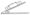Or

Determine the constant force P that will give the system of bodies shown , a velocity of 3m/sec after moving 4.5 m from rest.

Coefficient friction between the blocks and the plane is o.3 . Assuming pulley are smooth.### 1 thought on “CSVTU Exam Question Papers-BE First-Year-Engineering Mechanics–Nov-Dec-2006”

1.Exam paper 2016